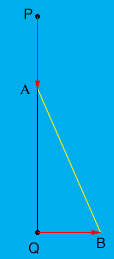SEARCH HOMEMath Central Quandaries & QueriesQuestion from Gevork, a student: Ship A is sailing due south at 16 mph. At the same time, a second ship B, 32 miles south of A, is sailing due east at 12 mph. (a) at what rate are they approaching or separating at the end of one hour? (b) At what rate are they approaching or separating at the end of two hours? (c) When do they cease to approach each other and how far apart are they at that instant.Gevork,

Draw a diagram.

 P is the position of A and Q is the position of B at time zero. At time $t$ hours ship A is at position A and ship B is at position B. Since ship A travels at 16 mph the distance from P to A is $16 t$ miles. What is the distance from Q to B? What is the distance from A to Q? Use Pythagoras' theorem to find the distance from A to B as a function of time $t.$ The derivative of this function with respect to $t$ tells you the rate at which the distance from A to B is changing at time $t$ hours.PennyMath Central is supported by the University of Regina and The Pacific Institute for the Mathematical Sciences.